Programming Truth Table reductionClick [ HERE ] for an introduction to Boolean Algebra.
Click [ HERE ] to go to the Logic10 page.

Introduction

Logic10 is a Windows program for applications of Boolean Algebra.
It's purpose is to :
- generate Truth Tables from formula's
- reduce Truth Tables to the simplest form.

Boolean Algebra

Like any algebra, Boolean Algebra has variables and operators.

Variables

Are denoted by a single capital letter: A, B, C......
A variable can have the value true (1) or false (0).

Operators

. for AND
+ for OR
/ for inverse
Note: Normally, the inverse oparator is a horizontal bar on top of the character however, this is hard to edit.
" / " has the highest priority, then " . " , then " + "

Note: Between variables, the " . " operator may be omitted, so AB = A.B

Formula's

Variables and operators together make formula's.
The most convenient format is CNF ( conjunctive normal form).
This is the format
ABC + DEFG + H + IJ
ABC, DEFG, H , IJ are called terms.

CNF is a list of terms that are OR'ed.

The CNF formula AB + /AC is true if A=1 and B=1........OR..........if A=0 and C=1

Data Structures

In Logic10, a maximum of 15 variables is allowed.
These characters are stored in alphabetic order in the Variable Table.

In a formula, a variable may be in the true or in the false state.
In AB/CD , A,B,D are in true- , C is in false (negated) state.
Terms are coded in a 16 bit word.
Each bit (0..14) corresponds with a variable in the Variable Table.
A bit is 0 for the variable in the negated state, a bit is 1 for the variable in the true state.
So, the term is true when it's bits are the same as the variable values.
Below is the term: A/BCD/E/FGH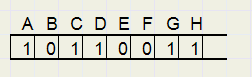In formula AB/C + ADCE + D/E we notice variables A,B,C,D,E.
Each term holds only some variables, not all.
So per variable a bit is needed to indicate the presence.
Another 16 bit word holds bits that enable (1) or disable (0) the corresponding variable.
Below is pictured the term /BCEF/G where the Variable Table is ABCDEFGH.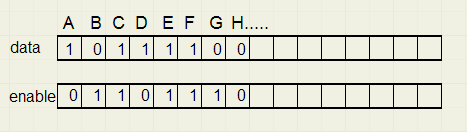Two 16 bit words are needed per term.
A term is the AND of the enabled variables in true or false state.

The Truth Table consists of 3 arrays:
```TTD : array[1..32768] of word ..........data terms
TTE : array[1..32768] of word...........enables
TTF : array[1..32768] of boolean
```
Note: 32768 = 215
The TTF array purpose is explained later.
It was added in version 2.0

Reduction Rules

Reducing a truth table amounts to the elimination of redundant variables in terms or the deletion of a complete term.
So, only TTE[ ] entries are modified during reduction.
The following rules of Boolean Algebra are applied:
 1 A + A = A absorbtion law 2 A + AB = A extra absorbtion law 3 AB + A/B = A single difference law 4 A + /AB = A + B complement extra absorbtion law 5 /AB + AC ---> BC virtual term generation
Note: the name "absorbtion law" is found in technical literature, but the additions "extra" , "complement" or
"virtual term", are my own.

Scanning the truth table

Reducing the truth table requires that each entry of TTD,TTE is compared to all other entries.
As indexes to TTD,TTE, variables i , j are used, where i < j.
So TTD[ i ] is compared to TTD[ j ] and TTE[ i ] is compared to TTE[ j ].
A complete scan requires i to increment from 1 to topentry-1 and j to count from i+1 to the topentry of TTD,TTE.

In case of a change during the scan, a rescan takes place until a complete scan yields no changes any more.
For rule 5, an extra scan using index k, takes place to compare the virtual term with all terms in the truth table.

Analyses

Variables

These variables play a role in truth table reduction:

The Vmask word variable has a 1 bit set for each variable in the Variable Table.
So, if the Variable Table holds P,Q,V,W,Z, then vmask is 11111 binairy (\$1f hexadecimal) , one bit for each variable.

2. diff
Diff is an abbreviation of difference, this is the exclusive or between TTD[ i ] , TTD[ j ].
```diff := (ttd[i] xor ttd[j]) and (tte[j] and tte[i]);
```
Only variables that appear in both terms are compared.
Picture below compares /AC to /A/BCD and finds they are equalReduction is based on the conditions hi , hj, sb , zb

hole-i (hi)

"Hole-i" means, that TTE[ i ] bit n = 0 and TTE[ j ] bit = 1.
Say TT[ i ] is A and TT[ j ] is AB : the B is missing in TT[ i].
Boolean variable hi = true in this case.
Code:
```   hi := ((vmask xor tte[i]) and (tte[j])) <> 0;
```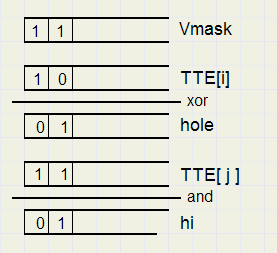hole-j (hj)

"Hole-j" means, that TTE[ j ] bit n = 0 and TTE[ i ] bit n = 1.
Boolean variable hj = true in this case.
Code:
```   hj := ((vmask xor tte[j]) and (tte[i])) <> 0;
```

sb (single bit)

If variable diff has only 1 bit set, then sb is true.
```   sb := SINGLEBIT(diff);
```
SINGLEBIT(v ) is a function that returns true if only 1 bit in word v is set (1).

Zero (zb)

The zero flag zb is set if diff = 0.
Terms A/BPQR and A/BVWX are equal, diff will be 000000000 because their common part A/B is equal.
/ABCD and ABCD are not equal, because A and /A differ.

Vmask, diff, hi, hj, sb, zb provide all information necessary for truth table reduction.

Reduction for laws 1..4

The conditions: hi,hj,sb,zb are encoded: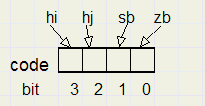Rule 1 : Absorbtion Law

Code = 1 ( hi=0, hj=0 sb=0 zb=1).
Action: set TTE[ j ] := 0;

Rule 2 : Extra Absorbtion Law

1. code = 5 (hi=0 , hj=1 , sb=0, zb=1)
Action: set TTE[ i ] = 0

2. code = 9 (hi=1, hj=0, sb=0,zb=1)
Action: set TTE[ j ] = 0.

So, either term [ i ] or term [ j ] is deleted.

Rule 3 : Single Difference Law

Code = 2 (hi=0, hj=0, sb=1, zb=0)
Action: set TTE[ j ] = 0, delete single difference bit in TTE[ i ]
```  tte[n] := tte[n] and (vmask xor diff);
rescan := true;
```
The rescan flag causes a complete new scan after the current one is finished.

Example: P/QVZ + P/Q/VZ = P/QZ .
Assume variable table = 'PQVZ';And variable V is eliminated.

Rule 4 : Complement Extra Absorbtion Law

code 6 (hi= 0 hj=1 sb=1 zb=0)
Example [ i ] AB + [ j ] /B = [ i ] A + B , where [ j ] has a hole and 1 variable (B) is different.
Action: Delete [ i ] difference.

code 10 ( hi=1 hj=0 sb=1 zb=0)
Example [ i ] PQRST + [ j ] PQR/STU = [ i ] PQRST + [ j ] PQRTU, where [ i ] has a hole (missing U) and S is different.
Action: Delete [ j ] difference.

Rule 5 : Virtual terms

Reduction laws 1..4 do most of the work, but not all.
The idea of virtual terms came up after I noticed that formula's like AB + /AC may generate an extra term BC.
BC is not wrong, but redundant.
If BC = 1 then also AB + /AC must be 1, but the opposite is not true.
So BC is a kind of representation of AB + /AC which I called the parents of BC.

So, if rules 1..4 do not apply, a virtual term may show up in the reduction process.
This virtual term is then compared to all other terms in the truth table using index [ k ].
A virtual term may reduce other terms and also itself with one exception:
virtual terms may not delete their parents.
So registration is needed for each entry in the truth table for being a parent.
The TTF boolean array serves this purpose.
If TTF[ k ] is true, then TTD,TTE[ k ] is a parent of the current virtual term.
The following examples have the virtual term printed fat and the parent printed red
AB + AB = no action
AB + ABC = AB + AB
AB + ABC = AB
AB/C + ABC = AB + AB
Note: if the virtual term is reduced, the truth table entry [ k ] becomes a parent.
This prevents elimination in subsequent scans.

If the virtual term is modified, virtual scanning restarts at k = 1.

A virtual term is recognized by code = 14 (hi = 1, hj = 1, sb = 1 , zb = 0).
The single difference bit is left out, the other (equal) variables are united.
The virtual term has variable VTE for the enables and VTD for the data.
```//  ------   ABC + /ABD ----> virtual term BCD

VTE := (TTE[i] or TTE[j]) and (vmask xor diff);
VTD := ((TTD[i] and TTE[i]) or (TTD[j] and TTE[j])) and VTE;
TTF[i] := true;
TTF[j] := true;
```

Virtual term Stack

```type TV = record         //virtual term
d : word;     //data
e : word;     //enables
p : word;     //parent
end;

const vstacklength = 15;
var
VStack : array[1..vstacklength] of TV; //virtual terms stack
vwrite : byte;        //write pointer for Vstack
```
During the compare of a virtual term with terms in the truth table, a new virtual term may be generated.
This new virtual term is stored in a stack (actually FIFO buffer) for later compare.
The "p" field in the stack entry holds one parent which is an extra (third or more) parent.
A virtual term emerges by a certain difference bit, a variable that appears in both normal and complemented form
of the terms being OR'ed during the scan.
The stack holds only one entry per (different) variable.
Variable Vhist holds the difference bits of the stack entries and prevents that duplicate virtual terms are stored.
So for the stack, a length of 15, the maximal number of variables, suffices.

Control

Table reduction may take some time, when many variables are involved.
(maximum time measured is about 30 secs)
To keep control and receive a lifesign, the scanning is interrupted after 250000 compares
and control is given to the calling procedure.
It is the responsibility of this procedure to display a lifesign and restart the scanning process.

Function TTCompare( scanmode: TReduceproc) : TReduceproc; performs the truth table reduction
and exits after 250000 compares, with result ttPause.

Procedure Reduce calls TTCompare and takes care of the generation of a lifesign.

The continueflag is cleared by pressing the ESCAPE key, which terminates the process.
```type TReduceProc = (ttStart,ttPause,ttEnd);
....
procedure Reduce;
const s1 = 'scanning truth table ';
var scancount,n : byte;
scanmode : TreduceProc;
s : string;
begin
scancount := 1;
scanmode := ttStart;
form1.msglabel.caption := s1;
application.processmessages;
repeat
scanmode := TTcompare(scanmode);
s := s1;
for n := 1 to scancount do s := s + '>';
form1.msglabel.caption := s;
application.processmessages;
inc(scancount);
if scancount = 10 then scancount := 1;
until (scanmode = ttEnd) or (continueflag = false);
if continueflag then TTcompress;
end;
```

Compression

At rescan time, when i = 1, j = 2, the truth table is compressed: deleted terms are removed by shifting
down valid entries.

Normal scan

Below is the flowchart of a normal scan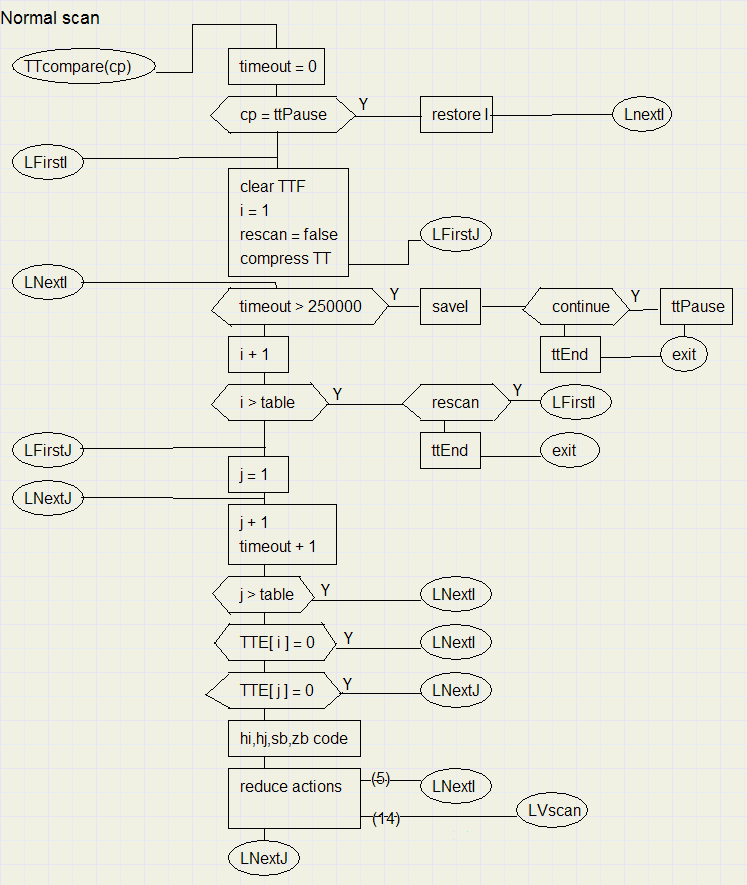The ovals are labels or program entries, exits.

Virtual scan

Below is the flowchart of a virtual scanVerification

Programming is making mistakes.
How can one be sure, that the result is correct?
A simple check for integrity is to evaluate the input (formula or table) for all values of the variables,
do the same for the reduced output table and compare the results.
The reduction may (in rare cases) not be 100%, but at least no error is introduced by a false elimination.
The procedure TTVerify, added in version 1.2 , still does this job in version 2.0

This concludes the description of Truth Table reduction by Logic10 version 2.0